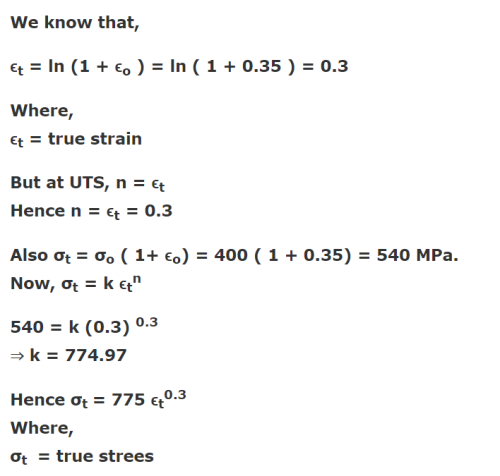Courses

# The ultimate tensile strength of a material is 400 MPa and the elongation up to maximum load is 35%. If the material obeys power law of hardening, then the true stress-true strain relation (stress in MPa) in the plastic deformation range is:a)σ = 540ε0.30b)σ = 775ε0.30c)σ = 540ε0.35d)σ = 775ε0.35Correct answer is option 'B'. Can you explain this answer?

## Mechanical Engineering Question

Shweta Gupta answered Jul 12, 2018Anita Parida answered Jul 12, 2018
Get value of true strain, den get true stress wid d given data using d usual traditional formula..den use d power law of hardening, where u wil put d value of strain hardening component equals to ur engineering strain(its for necking to occur after strain hardening), den from power law get value of K..den put d same value in power law..n get d answer..Sorry unable to upload d image of explanation bcz of this app issue..